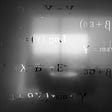# Euler Totient Function in its core!

## This blog aims to explain everything about the Euler Totient Function, covering every concept from its internal working(even the use case in number system) to its real-world applications in the field of Cryptography!

I am pretty sure that you all must have heard the name Euler at multiple locations with different contexts. Here also, there is one different context where Euler's name is there & it is there to provide one function or it can also be understood as a theorem that acts as a building block for multiple other theorems and areas.

This term “Euler Totient Function” is mainly related to the “Cryptography”. It can also be used in the number system concepts, that is, the function above can somehow help in some concepts in number system when it comes to the aptitude part.

# What is Euler Totient Funciton for?

The main concept with which the Euler Totient function deals is with the prime numbers. The main aim of it is to provide the total count of the numbers which are coprime/relative prime/mutually prime to a given number.

## What are coprime/relative prime/mutually Prime Numbers?

These are the numbers which are having their gcd(a, n) = 1, where “n” is the actual number, & at the place of “a” there can be multiple numbers. For Example, let n = 7. Now all those numbers which are having their gcd with n as 1, will be called as coprime/relative prime/mutually prime to m.

In the example, numbers which are coprime to n are: {1,2,3,4,5,6}.

Let us take n = 9, in this case coprime numbers are: {1, 2, 4, 5, 7, 8}.

## Notation of Euler Totient Function!

It is denoted by the “phi” symbol.

For any number “n”, Euler Totient Function will be represented as phi(n). It represents the total count of numbers which are coprime to n. In the above examples, where n = 7, there phi(n) = 6, & when n = 9, there phi(n) = 6.

## The shortcut of calculating the phi(n) in case of Prime numbers!

When the number is prime, then phi(n) = n -1 always. It can be verified by taking any prime number, let us take the above given example where n = 7, then phi(n) = 6, take n = 5, then coprime numbers to 5 are {1, 2, 3, 4}, which means phi(n) = n -1 = 5 -1 =4.

## Amazing Property of Euler Totient Function!

If the number whose phi(n) has to be calculated is very large, then there is a property to break that, & that property is given below.

The above image represents the property which is used to break the number when n is large. For example, if n = 3127, then it can be broken into 2 multiples that are 53 & 59.

Now, it is well known that 53, as well as 59 both, are prime numbers, then the aforementioned shortcut can be applied easily here to calculate the values of phi(53) as well as phi(59).

The values for phi(53) & phi(59) are 52 & 58 respectively.

## Use-Cases of the Euler Totient Function!

It is heavily used in Cryptography various algorithms & Methods. It is even the base for the algorithms to work in Cryptography.

Some of the example algorithms where it is applied are:

• Euler/Euler-Fermat Theorem.
• Fermat's Theorem.
• RSA

The above mentioned are few examples of its use-case. Although it can be used in various situations in number system also, where count of coprime numbers are to be found for a number.

I hope my article explains each and everything related to the topic with all the deep concepts and explanations. Thank you so much for investing your time in reading my blog & boosting your knowledge. If you like my work, then I request you to give an applaud to this blog!

--

--

--

## More from Analytics Vidhya

Analytics Vidhya is a community of Analytics and Data Science professionals. We are building the next-gen data science ecosystem https://www.analyticsvidhya.com

## Avast Ye!## Heuristic Solutions## Graphs, graphs everywhere!## Error Intervals## Essential Math for Data Science## Chemical Kinetics & Rate of Production: An Interesting Analogyg## But what is a quantum phase factor?## Harshit Dawar

Big Data Enthusiast, have a demonstrated history of delivering large and complex projects. Interested in working in the field of AI and Data Science.

## Recommendation System with Apache Beam as the Streaming Data-Parallel Processing Pipeline